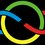# International Mathematical Olympiad 60', Second Day

The goal of this set of notes is to improve our problem solving and proof writing skills. You are encouraged to submit a solution to any of these problems, and join in the discussion in #imo-discussion on Saturday 24 at 9:00 pm IST ,8 30 PDT. For more details, see IMO Problems Discussion Group.

Sorry Everyone!, I was really busy the last whole week. Here is the next set of problems from the 1960 IMO.

Second Day

4. (HUN) Construct a triangle $ABC$ whose lengths of heights $h_a$ and $h_b$ (from A and B, respectively) and length of median $m_a$ (from A) are given.

5. (CZS) A cube ABCDA'B'C'D' is given.

(a) Find the locus of all midpoints of segments $XY$, where $X$ is any point on segment $AC$ and $Y$ any point on segment B'D'.

(b) Find the locus of all points $Z$ on segments $XY$ such that $\vec{ZY} = 2 \vec{XZ}.$

6. (BUL) An isosceles trapezoid with bases $a$ and $b$ and height $h$ is given.

(a) On the line of symmetry construct the point P such that both (nonbase) sides are seen from P with an angle of $90^{\circ}$.

(b) Find the distance of $P$ from one of the bases of the trapezoid.

(c) Under what conditions for $a, b$, and $h$can the point $P$ be constructed (analyze all possible cases)?

7. (GDR) A sphere is inscribed in a regular cone. Around the sphere a cylinder is circumscribed so that its base is in the same plane as the base of the cone. Let $V_1$ be the volume of the cone and $V_2$ the volume of the cylinder.

(a) Prove that $V1 = V2$ is impossible.

(b) Find the smallest $k$ for which $V_1 = k V_2$, and in this case construct the angle at the vertex of the cone.

###### This is part of the set International Mathematical OlympiadsNote by Sualeh Asif
5 years, 7 months ago

This discussion board is a place to discuss our Daily Challenges and the math and science related to those challenges. Explanations are more than just a solution — they should explain the steps and thinking strategies that you used to obtain the solution. Comments should further the discussion of math and science.

When posting on Brilliant:

• Use the emojis to react to an explanation, whether you're congratulating a job well done , or just really confused .
• Ask specific questions about the challenge or the steps in somebody's explanation. Well-posed questions can add a lot to the discussion, but posting "I don't understand!" doesn't help anyone.
• Try to contribute something new to the discussion, whether it is an extension, generalization or other idea related to the challenge.
• Stay on topic — we're all here to learn more about math and science, not to hear about your favorite get-rich-quick scheme or current world events.

MarkdownAppears as
*italics* or _italics_ italics
**bold** or __bold__ bold
- bulleted- list
• bulleted
• list
1. numbered2. list
1. numbered
2. list
Note: you must add a full line of space before and after lists for them to show up correctly
paragraph 1paragraph 2

paragraph 1

paragraph 2

[example link](https://brilliant.org)example link
> This is a quote
This is a quote
    # I indented these lines
# 4 spaces, and now they show
# up as a code block.

print "hello world"
# I indented these lines
# 4 spaces, and now they show
# up as a code block.

print "hello world"
MathAppears as
Remember to wrap math in $$ ... $$ or $ ... $ to ensure proper formatting.
2 \times 3 $2 \times 3$
2^{34} $2^{34}$
a_{i-1} $a_{i-1}$
\frac{2}{3} $\frac{2}{3}$
\sqrt{2} $\sqrt{2}$
\sum_{i=1}^3 $\sum_{i=1}^3$
\sin \theta $\sin \theta$
\boxed{123} $\boxed{123}$

## Comments

Sort by:

Top Newest

@Xuming Liang @Nihar Mahajan here is the whole load of Olympiad Geometry! Enjoy!

- 5 years, 7 months ago

Log in to reply

Sketch for a brutal solution for $4$.

From $h_a,m_a$ we can construct the vertex $A$, its foot of perpendicular on and midpoint of $BC$, which are denoted $D,M$ respectively. From $h_a,h_b$ we know the ratio of $AC,BC$ and $MC,AC$; specifically $\frac {MC}{AC}=\frac {h_b}{2h_a}$. Thus we can construct $C$ with the use of Apollonius circle, and $B$ consequently follows.

- 5 years, 7 months ago

Log in to reply

My solution to Q4:

Construction: Draw segment $AM$ with length $M_a$. Now, draw a circle centred at $A$ with radius $h_a$. Draw one of the tangents of this circle passing through $M$. Now construct a circle with radius $\dfrac {h_b}{2}$ centred at $M$. Draw one of the tangents of this second circle passing through $A$. The intersection of these two tangents is $C$. Draw a line parallel to the second tangent with a distance of $h_b$ between them. The intersection of this line and the firs tangent is $B$. Thus, we have the 3 points $A$, $B$ and $C$ to construct triangle $ABC$.

Explanation: In a triangle $ABC$, points $M$ and $N$ are the midpoints of sides $BC$ and $AC$ respectively. We have that $\Delta MNC \sim \Delta ABC$ and as a result point $M$ is a distance of $\dfrac {h_b}{2}$ away from $AC$. The rest is self-explanatory.

- 5 years, 7 months ago

Log in to reply

×

Problem Loading...

Note Loading...

Set Loading...